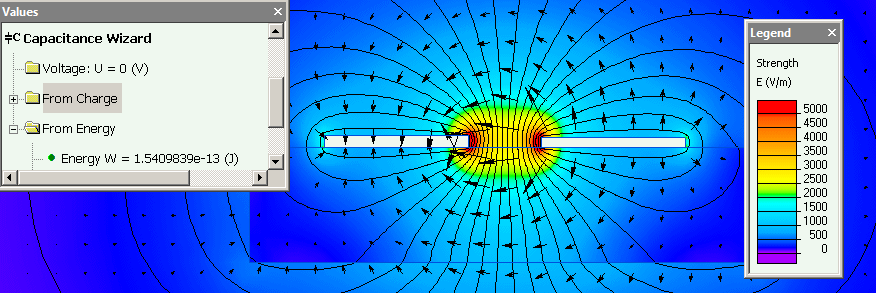Main >> Applications >> Sample problems

# Interdigital capacitor

interdigitated capacitor capacitance, PCB capacitor, thin film capacitor, interdigital capacitor model

This is an example of the interdigital capacitor simulation, performed with QuickField software in both 2D and 3D formulations.

Problem Type
Plane-parallel problem of Electrostatics,
3D extrusion model of Electrostatics.

Geometry

Trace thickness 40 μm. Board thickness 0.4 mm.

Given
Relative permittivity of the FR4 dielectric ε = 4.7;
The voltages applied to capacitor plates are -0.5 and +0.5 V.

Determine the self-capacitance of the interdigital capacitor.

Solution
Interdigital capacitor is designed to be made by the microstrip lines on the PCB. Its capacitance is defined by the air gap between the electrodes. To correctly simulate the interdigital capacitor we need a full 3D analysis.
However, for brief evaluation of the capacitance we may use 2D approach. We can virtually straighten the capacitor and calculate the capacitance between two planar electrodes in 2D. As the length of the model, we take the length of the mid-line along the capacitor gap, which equals to L = 7250 micrometers.
Capacitor capacitance in both 2D and 3D formulations can be obtained from the energy of the electric field W and the potential difference U:
C = 2W / U²

Results
Interdigital capacitor capacitance in 2D: C = 2 * 1.54e-13 / 1²= 0.31 pF.Interdigital capacitor capacitance in 3D: C = 2 * 1.27e-13 / 1² = 0.25 pF.• Download simulation files (files may be viewed using any QuickField Edition).
•